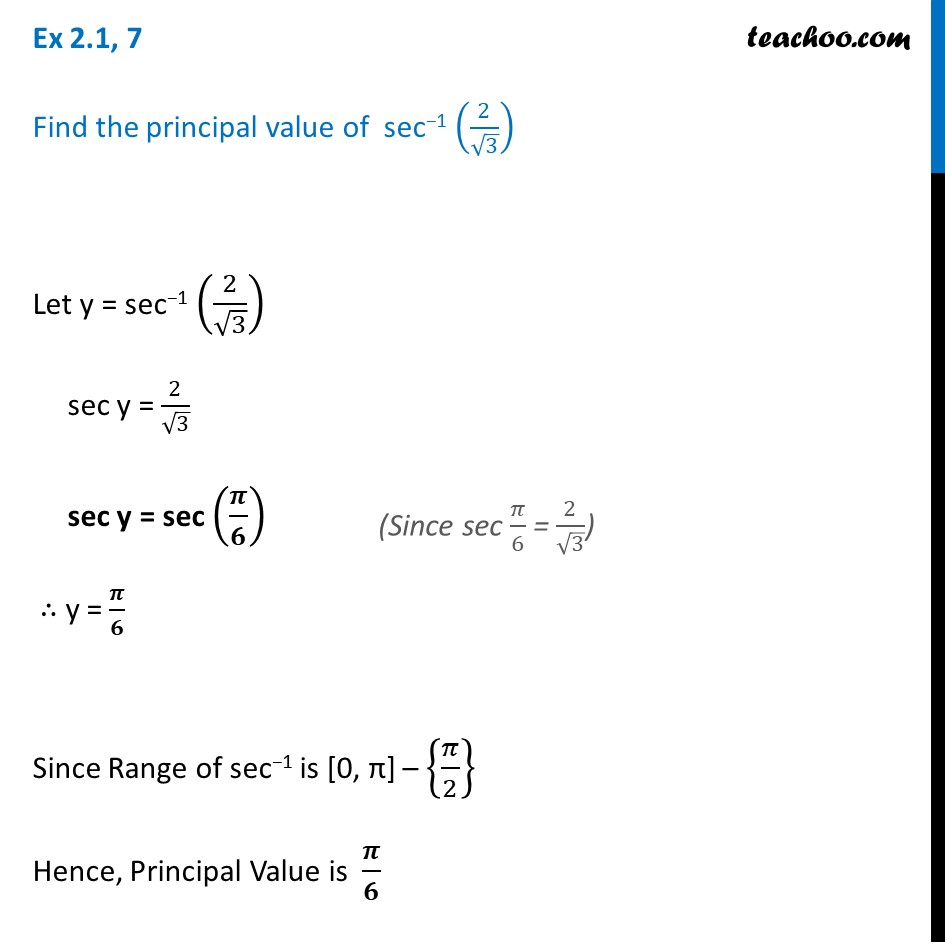1. Chapter 2 Class 12 Inverse Trigonometric Functions (Term 1)
2. Serial order wise
3. Ex 2.1

Transcript

Ex 2.1, 7 Find the principal value of sec−1 (2/√3) Let y = sec−1 (2/√3) sec y = 2/√3 sec y = sec (𝝅/𝟔) ∴ y = 𝝅/𝟔 Since Range of sec−1 is [0, π] – {𝜋/2} Hence, Principal Value is 𝝅/𝟔 (Since sec 𝜋/6 = 2/√3)